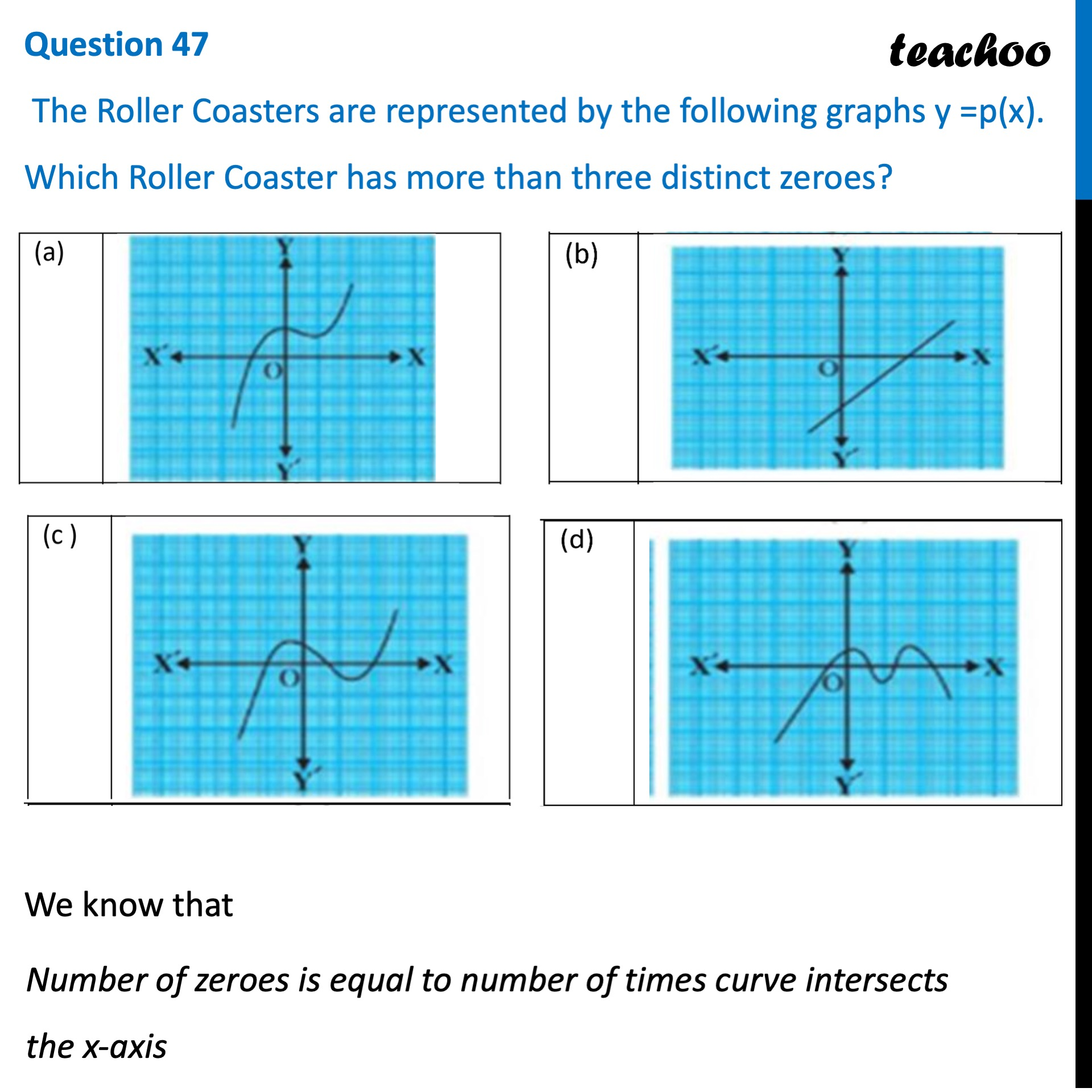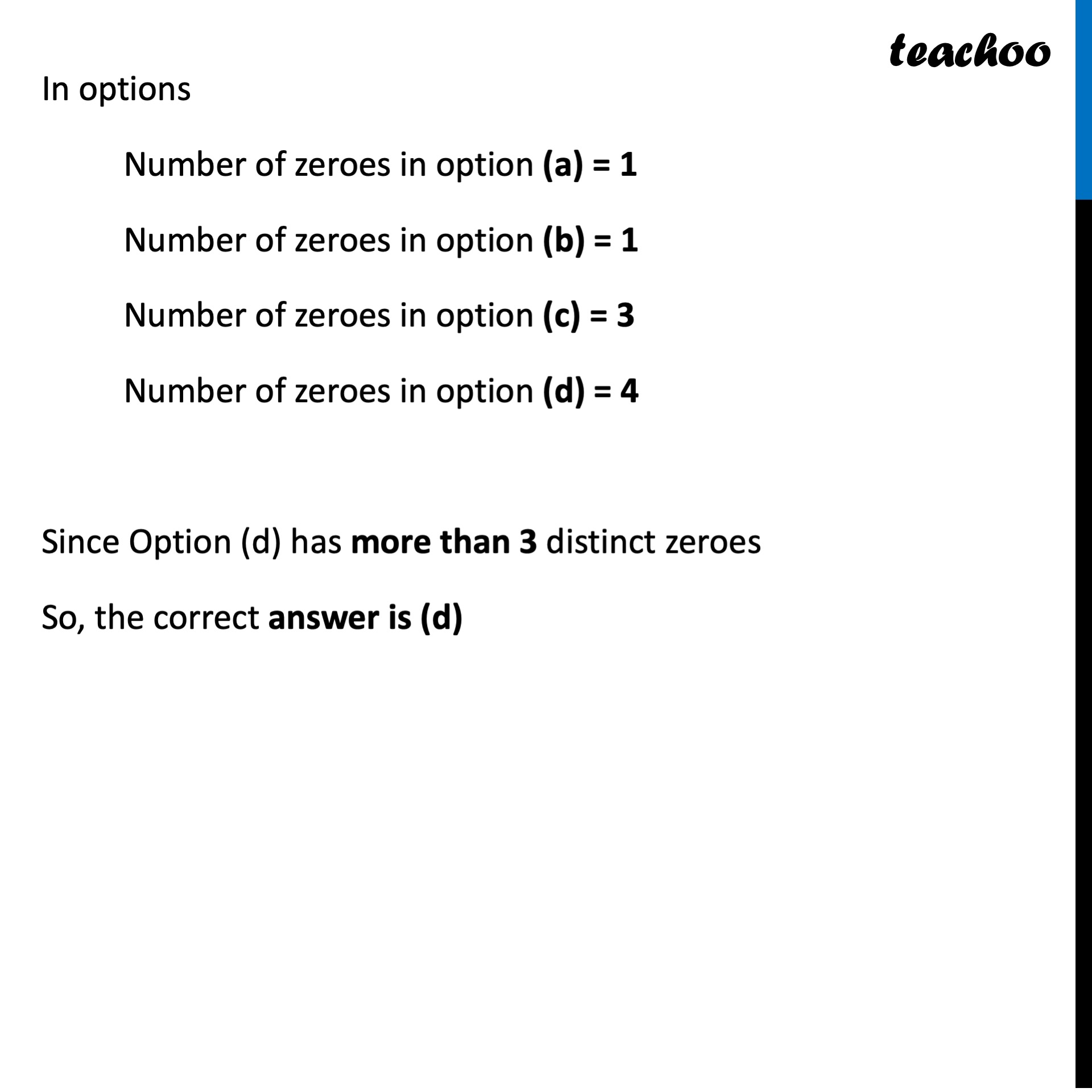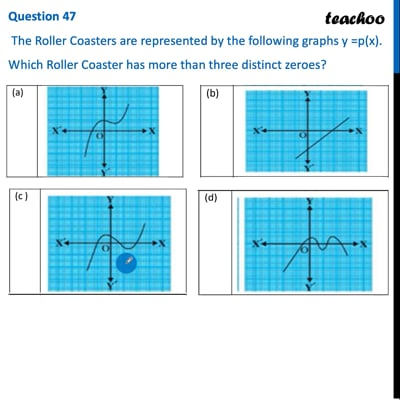CBSE Class 10 Sample Paper for 2022 Boards - Maths Basic [MCQ]

Class 10
Solutions of Sample Papers for Class 10 Boards

## The Roller Coasters are represented by the following graphs y =p(x). Which Roller Coaster has more than three distinct zeroes?This video is only available for Teachoo black users

Introducing your new favourite teacher - Teachoo Black, at only ₹83 per month

### Transcript

Question 47 The Roller Coasters are represented by the following graphs y =p(x). Which Roller Coaster has more than three distinct zeroes? We know that Number of zeroes is equal to number of times curve intersects the x-axis In options Number of zeroes in option (a) = 1 Number of zeroes in option (b) = 1 Number of zeroes in option (c) = 3 Number of zeroes in option (d) = 4 Since Option (d) has more than 3 distinct zeroes So, the correct answer is (d)